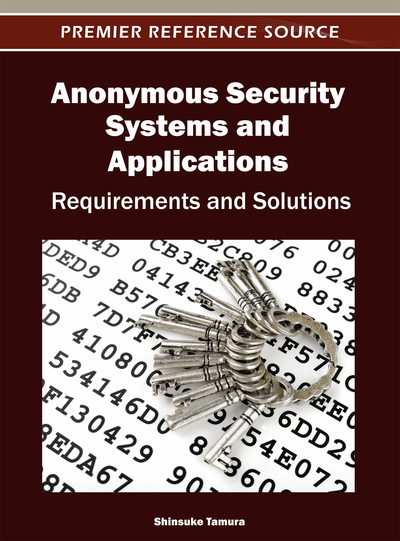# Anonymous Statistics Calculation

DOI: 10.4018/978-1-4666-1649-3.ch008

## Abstract

This chapter introduces schemes for anonymous statistics calculations, in which an entity or a set of entities calculate functions of data owned by other entities without knowing their values. Although schemes that can be applied to calculate general functions exist, they are not practical enough, therefore schemes applicable only to limited number of functions, e.g. averages, variances, auto-, and cross-correlations are discussed. They are blind sum/product calculation schemes, partial computation based multi party computation schemes, and re-encryption based multi party computation schemes.
Chapter Preview
Top

## Introduction

Let us consider a case where entity S calculates the average of salaries of persons P1, P2, ---, PN. In this case, usually salaries of persons are their secrets and they do not want to disclose them to others. Therefore mechanisms, which enable S to calculate the average of salaries without knowing those of individual persons, are necessary. Theoretically, there are protocols that enable N entities Q1, Q2, ---, QN to jointly calculate any function f(x1, x2, ---, xN) while maintaining values of x1, x2, ---, xN as secrets of their owners Q1, Q2, ---, QN (Goldreich, 1987, Yao, 1986). Then, entity S in the above case, can know the average of salaries while preserving privacies of P1, P2, ---, PN, by asking each Pj to carry out these protocols. However, these protocols are complicated and as a consequence cannot be used in real applications. Although efficient protocols exist, they are applicable only when f(x1, x2, ---, xN) is a Boolean expression (Naor, 1999). As practical schemes, this chapter discusses mechanisms based on encryption functions with the homomorphic property. But it must be noted that they are efficient only for limited kind of anonymous statistics calculations.

In the following, the above requirement is satisfied through 3 ways. The 1st way is the blind sum/product calculation scheme, the simplest one. There are 2 entities P and Q, where P encrypts its data x1, x2, ---, xN to E(kP, x1), E(kP, x2), ---, E(kP, xN) by its encryption key kP, and Q calculates f(E(kP, x1), E(kP, x2), ---, E(kP, xN)) to be decrypted to f(x1, x2, ---, xN) by P that knows decryption key kP-1. Then, P can calculate f(x1, x2, ---, xN) without disclosing its owning data, and on the other hand, Q can conceal function f. Here, function f(x1, x2, ---, xN) must have the specific forms, e.g. f(x1, x2, ---, xN) = x1+x2+ --- +xN, f(x1, x2, ---, xN) = x1x2 --- xN, etc. of course.

The 2nd and the 3rd ones are general multi party computation schemes, where multiple entities P1, P2, ---, PN jointly calculate f(x1, x2, ---, xN) while maintaining the secrets of their owning values x1, x2, ---, xN. In the 2nd way, each entity Pj that owns xj (j = 1, 2, ---, N) divides E(kC, xj) (here, encrypted form E(kC, xj) calculated based on publicly known key kC is a representation of xj) into H-parts E(kC, xj)1, E(kC, xj)2, ---, E(kC, xj)H so that no one can know xj without knowing all H-parts and anyone that knows kC can reconstruct xj when it knows all H-parts, and distributes individual parts E(kC, xj)1, E(kC, xj)2, ---, E(kC, xj)H to H-entities Qw1, Qw2, ---, QwH selected from Q1, Q2, ---, QN, respectively. Then, each Qwh calculates f(E(kC, x1)h, E(kC, x2)h, ---, E(kC, xN)h), and finally, coordinating entity C gathers calculation results of Qw1, Qw2, ---, QwH to calculate f(E(kC, x1), E(kC, x2), ---, E(kC, xN)), and from that f(x1, x2, ---, xN) is reconstructed, where it is assumed that relation E(kC, f(x1, x2, ---, xN)) = f(E(kC, x1), E(kC, x2), ---, E(kC, xN)) is satisfied.

## Complete Chapter List

Search this Book:
Reset